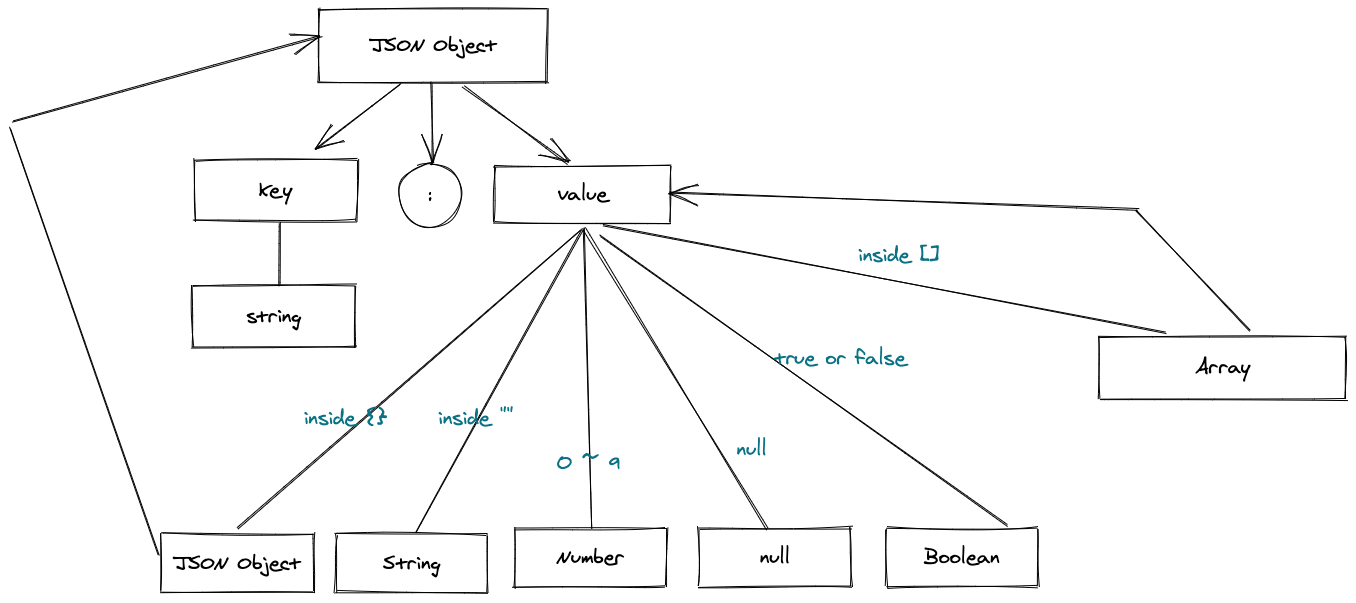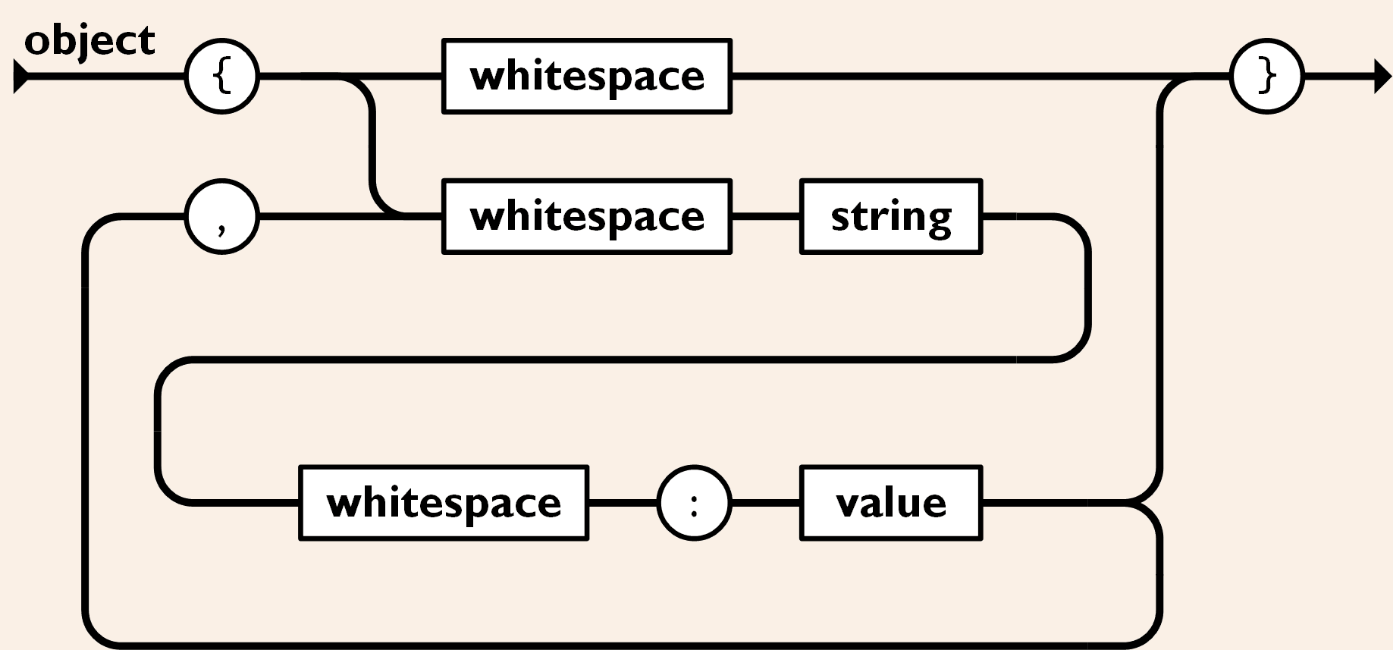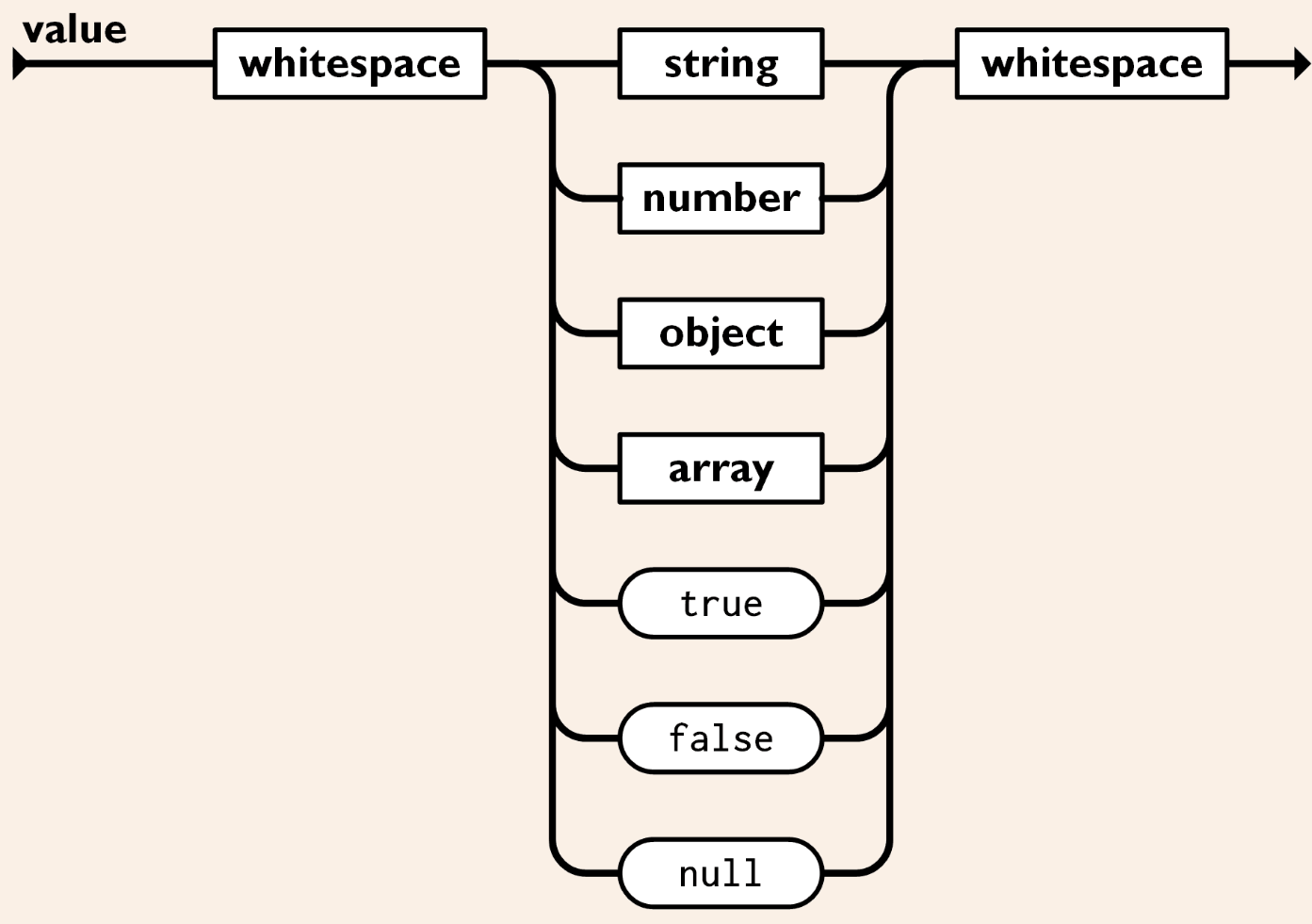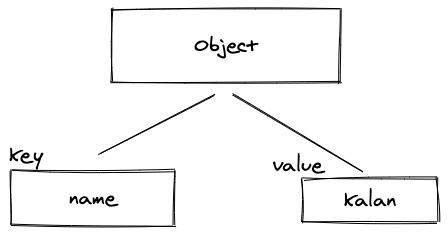# Kalan's Blog# 從無到有寫一個 JSON 解析器（1）

## 為什麼要學遞迴下降？

{
"name": "kalan",
"age": 20
}

{
"name": "kalan",
"age": 20,
"job": $job$
}

parse(json, { job: 'engineer' });

// {
//   "name": kalan,
//   "age": 20,
//   "job": "engineer"
// }

{
"name" @ "kalan"
"age" @ 20
}

## 遞迴下降（Recursive Descent）是什麼？{
"name": "kalan"
}• 遇到 { ，開始 object 解析
• 進入 key 解析，預期為字串
• 遇到 " ，字串解析
• 遇到 n, a, m, e：將 name 當作 key
• 遇到 " ：結束字串匹配，預期會有 : 以符合規則
• 遇到 : ：進入 value 解析
• 遇到 " ：進入字串解析
• 遇到 k, a, l, a, n：將 value 設為 kalan
• 遇到 "：結束字串匹配
• 遇到 }：結束 object 匹配
• 遇到空字串（EOF）：結束解析

## 一個簡單的解析器實作

class Parser {
constructor(raw) {
this.raw = raw;
this.index = 0;
}

// 目前字元
current() {
return this.raw[this.index];
}

// 匹配之後的字串是否為 str
// e.g: next('{')
// 判斷下一個字元是否為 '{'
// 如果是的話將 index 移過去
// 並且回傳 true
// 方便判斷狀態
next(str) {
if (this.raw.slice(this.index, this.index + str.length) === str) {
this.index += str.length;
return true;
}

return false;
}

// 省略空白，目前只考慮 " "
// 不實作 tab 等功能
skip() {
while (this.raw[this.index] <= " ") {
this.index += 1;
}
}

parse() {
const value = object(this); // entry point
return value;
}
}

function object(parser) {
const obj = Object.create(null);
let key = "";
// 如果是 { 開頭，代表要用 object 函數解析
if (parser.current() === "{") {
parser.next("{");
parser.skip();

// 如果馬上遇到 }
// 代表他是一個空物件 {}
if (parser.current() === "}") {
parser.next("}");
return obj;
}

// 讀取當前字元，判斷要用哪一個函數解析
while (parser.current()) {
key = string(parser); // key 一定是字串，所以我們用字串解析

parser.skip(); // 省略空白字元
parser.next(":"); // 預期是 :
obj[key] = string(parser); // 先假設值只有字串，一律用字串解析
parser.skip();
if (parser.current() === "}") { // 如果遇到 }，代表物件解析結束，return obj
parser.next("}");
return obj;
}
parser.next(","); // 如果有逗號，代表有下一個 key-value，繼續跑 while 解析
parser.skip();
}
}
}
• 如果現在的字元是 {

• 如果下一個字元是 } ，代表是空物件，回傳後跳出
• 進入 while 迴圈，用 string 解析 key（因為 JSON 的 key 一定是字串）。 string 函數的實作我們下面講解。

• 省略空白字元（像下列情形）
{ "name"         : "value" }
• 預期 : 出現
• 將 value 用 string 解析（這邊我們先暫時假設只有字串，後面再陸續補上其他型別的處理）
• 省略空白字元
• 如果遇到 } 代表物件結尾，回傳 obj
• 如果遇到 , ，代表要繼續解析下一個 key-value
• 省略空白字元

function string(parser) {
parser.next('"'); // 如果下一個字元為 "，代表我們要用 string 的方式解析
if (parser.next('"')) {
// { "": "value" } 我們將 key 為空的 JSON 當做不合法的
throw new Error("JSON key is empty.");
}

let key = "";
let curr = "";
// 不斷讀取下一個字元直到讀到 "
while (((curr = parser.current()), curr)) {
if (parser.next('"')) {
parser.index += 1;
return key;
}
key += curr;
parser.index += 1;
}
}
• 預期字元為 "
• 如果下一個字元為 " 代表是個空字串，為不合法的 key，跳出警告
• 進入 whilte 迴圈直到讀到字元 "

## 結語

1. 為了讓錯誤訊息更友善，或許我們可以用 row, column 二維的方式記錄資訊而非一維。
2. 實作更友善的錯誤訊息，例如忘記加 } 的時候可以提醒開發者，或是語法錯誤時可以明確指出錯誤的地方